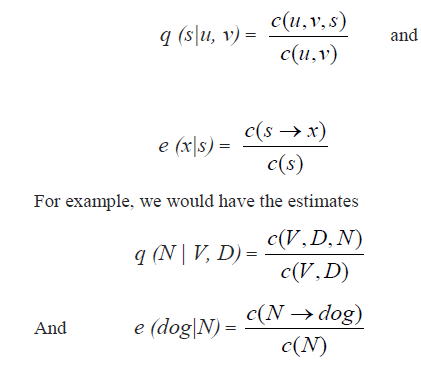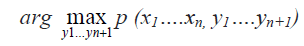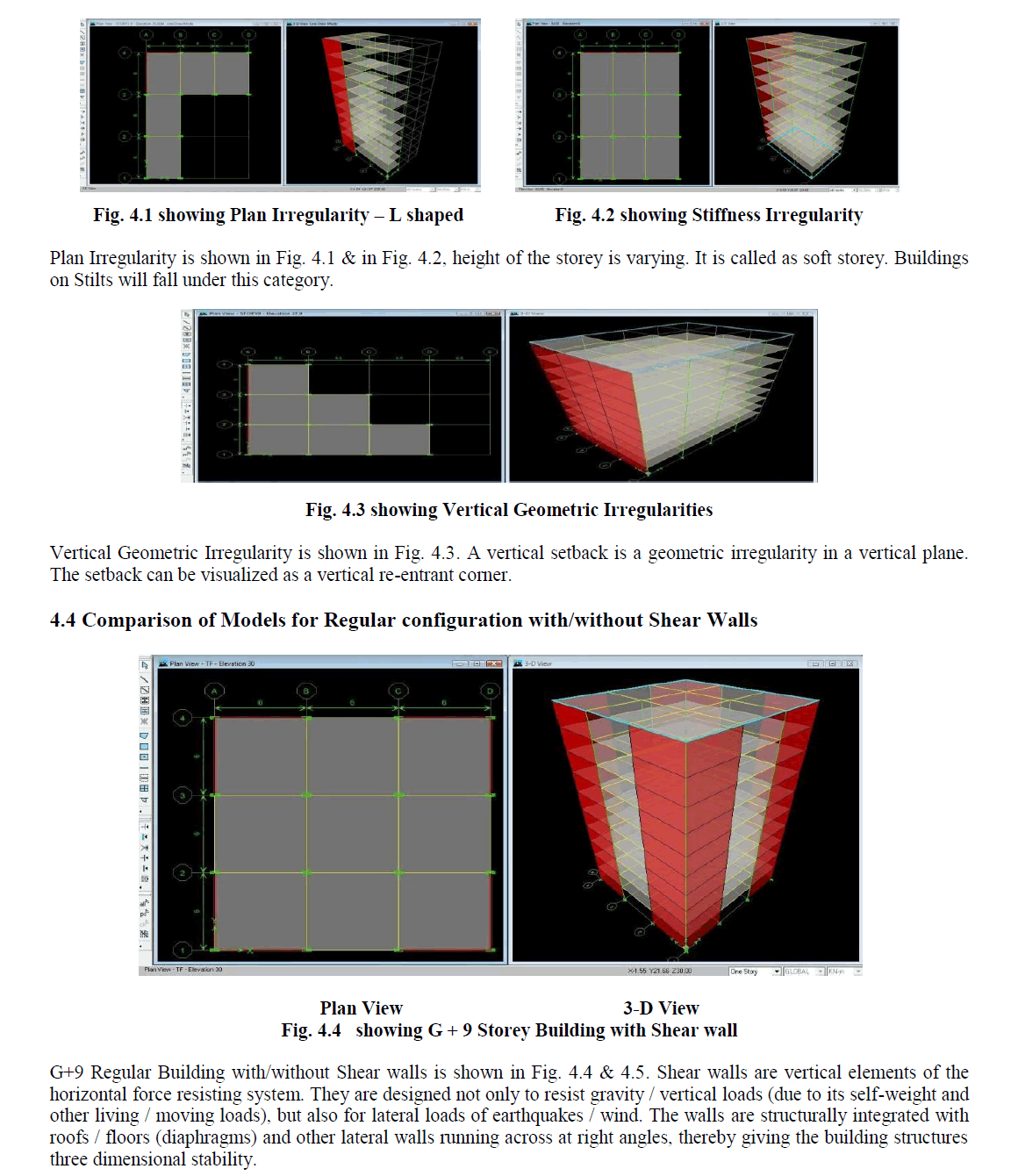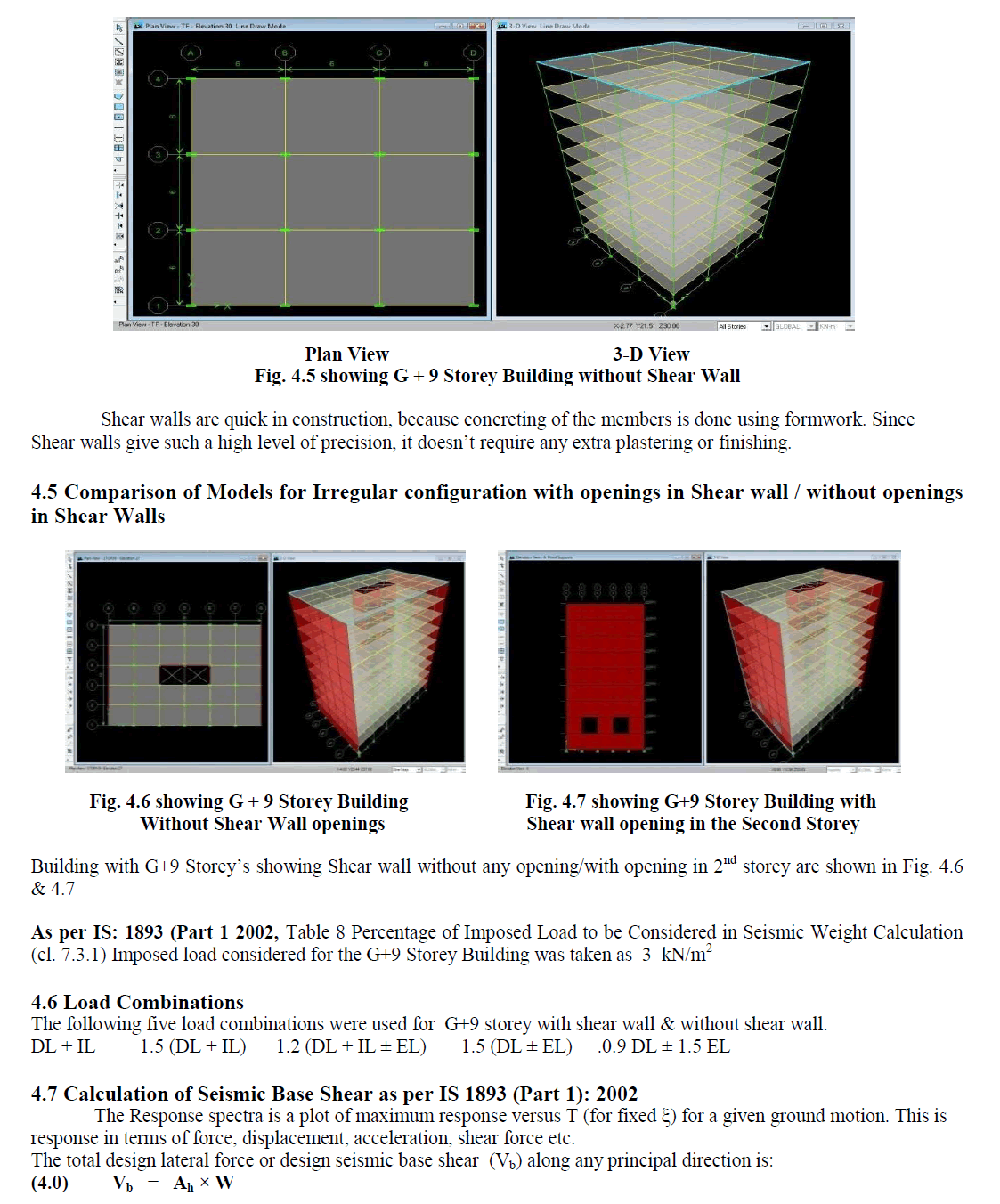ISSN ONLINE(2319-8753)PRINT(2347-6710)

All submissions of the EM system will be redirected to Online Manuscript Submission System. Authors are requested to submit articles directly to Online Manuscript Submission System of respective journal.

# A Trigram HMM Model For Solving Parts-of- Speech (PoS) Tagging Problems

 B.S.Uma 1, P.Penchala Prasad 2 P.G. Student, Department of Computer Science and Engineering, GPREC Engineering College, Kurnool, Andhrapradesh, India Assistant Professor, Department of Computer Science and Engineering, GPREC Engineering College, Kurnool, Andhrapradesh, India Related article at Pubmed, Scholar Google

Visit for more related articles at International Journal of Innovative Research in Science, Engineering and Technology

## Abstract

In the most of the Natural language processing problems, we have to model pair of sequences. The Parts Of Speech tagging (PoS) is the best solution for this type of problems. In POS tagging problem, our goal is to build a proper output tagging sequence for a given input sentence. The tag sequence is same as the input sequence. To get the POS tagging we have used the Hidden Markov Model (HMM) along with the Stanford POS parser in this paper.

### Keywords

HMM model, PoS Tagging, tagging sequence, Natural Language Processing.

### INTRODUCTION

In the corpus-linguistics, parts-of-speech tagging (POS) which is also called as grammatical tagging, is the process of marking up a word in the text (corpus) corresponding to a particular part-of-speech based on both the definition and as well as its context. In the olden days, it is used to be performed by hand, POS tagging is now done in the context of computational linguistics, using algorithms by associating discrete terms as well as hidden parts of speech, in accordance with a set of descriptive tags. POS-tagging algorithms fall into two distinctive groups: rule based and stochastic. Parts-of-speech tagging is harder than just having a set of words and their parts-of-speech, because certain words can be represented as more than one type of speech at the same time. A large percentage of the word-forms are ambiguous. For example, even dogs which are usually thought of as just a plural noun can also be a verb: “The sailor dogs the hatch” Appropriate grammatical tagging should reflect that dogs used here are Verb, not as plural noun. Analysis is used to infer that “sailor” and “hatch” implicate dogs as action applied to the object “hatch”.

### RELATED WORK

In the childhood we have been taught that there are 9 parts of speech in English: noun, article, adjective, preposition pronoun, adverb, conjunction and interjection. However there are many sub-categories. For nouns, the plural possessive and singular forms can be distinguished. In many languages words are also marked for their “case” (role as subject, object, etc...), grammatical gender, and so on; while verbs are marked for tense, aspect, and so on; while verbs are marked for tense, aspect ,and other things .Linguistics distinguish parts of speech to various fine degrees, reflecting a chosen “tagging system”.
In POS tagging the goal is to build a model whose input is a sentence, for example:
“the dog saw a cat”
and whose output is a tag sequence, for example D N V D N (where D represents determiner, N as noun and V as verb).The input to the tagging model is denoted by X1, X2 ,………..Xn. It is often referred as a sentence. In the above example the length n=5 and X1 = the, X2 = dog, X3 = saw, X4 = a, X5 = cat. The output of the tagging model is denoted by Y1, Y2….Yn .In the above example Y1 = D, Y2 = N, Y3 = V etc. This type of problem, where the task is to map a sentence to a tag sequence is oft en referred as sequence labeling problem.
We will assume that we have a set of training examples, (X (i); Y (i)) for i =1::: m, where each X (i) is a sentence and each Y(i) is a tag sequence. Our task is to learn a function that maps sentences to tag sequences from these training examples. To achieve this goal we have used Hidden Markov Model (HMM) for this alignment process.

### PROPOSED METHOD

The proposed method gives an approach of finding different parts-of-speech for a given input sequence. This is achieved by using
1) Trigram HMM model 2) Stanford parser.
Definition of trigram HMM
A trigram HMM consists of a finite set of V possible words, and a finite set K of possible tags, together with the following parameters:
ï· A parameter q(s|u,v)
for any trigram u,v,s such that s € k á´ {STOP} and u,v € V á´{ * }.The value for q(s|u,v), can be interpreted as the probability of seeing the tags immediately after the bigrams of u,v.
ï· A parameter e(x|s)
for any x € V, s € K. The value for e (x|s) can be interpreted as the probability of seeing observation x paired with state s.
Define S to be the set of all sequence / tag-sequence pairs (x1..........xn, y1..........yn) such that n>=0, xi € V for i=1…..n and yi € K for i=1…n and yn=STOP.Independence Assumptions in Trigram HMMs
Consider a pair of sequences of random variables X1…Xn, and Y1…Yn, where n is the length of sequences. We assume that each Xi can take any value in a finite set V of words. For example, V might be a set of possible words in English, for example V= {the, dog, saw, cat, laughs…} Each Yi can take any value in a finite set K of possible tags. For example, K might be the set of possible Part-Of-Speech tags for English, e.g. K= {D, N, V…..} The length n is itself a random variable – it can vary across different sentences but we will use a similar technique to the method used for modeling variable length Markov process.
Our task will be to model the joint probability P(X1 = x1... Xn = xn , Y1= y1…Yn = y1) for any observation sequence x1….xn paired with a state sequence y1…yn for any observation sequence x1...xn paired with a state sequence y1….yn, where each xi is a member of V and each yi is a member of K.
The following process is a stochastic one which generates sequence pairs y1…yn+1 , x1….xn :
1. Initialize i =1 and y0= y-1 = *.
2. Generate yi from the distribution q ( yi|yi-2 , yi-1 )
3. If yi= STOP then return y1…yi, x1…xi-1. Otherwise, generate xi from the distribution e (xi|yi), set i = i+1, and return to step 2.
Parameters of Trigram HMM
With the accessed training data which is containing a set of examples, where each example is a sentence x1…xn paired with tag sequence y1…yn. With these data we have estimated the parameters in the following way: Define C (u, v, s) to be the number of times the sequence of three states (u, v, s) is seen in training data: for example, C (V,D,N) would be the number of times the sequence of three tags V,D,N is seen in the training corpus. Similarly, define C (u,v) to be the number of times the tag bigram (u,v) is seen. Define C(s) to be the number of times that the state s is seen in the corpus. Finally define C (s→x) to be the number of times that the state s is seen paired with the observation x in the corpus: for example C (N→dog) would be the number of times dog is seen paired with the tag N.
Given these definitions the maximum-likelihood estimates areThus estimating the parameters of the model is simple, just read off counts from the training corpus, and then compute the maximum - likelihood estimates.
Decoding with HMMs: viterbi Algorithm
The main problem lies in finding the most appropriate tag sequence for an input sentence. This is the problem of findingWhere the argmax is taken over all sequences y1…yn+1 such that yi € K for i= 1…..n and yn+1= STOP.
The naive brute force method would simply enumerate all possible tag sequences y1….yn+1, score them under a function p, and takes the highest scoring sequence. For example, given the input sentence the baby crawls
and assuming the set of possible tags is K= { D,N,V},we have to consider all possible tag sequences
D D D STOP
D D N STOP
D D V STOP
D N D STOP
D N N STOP
D N V STOP
There are 33= 27 possible sequences in this case. However this method is inefficient for longer sentences. For an input sentence of length n, there are |k|n possible tag sequences. The exponential growth with respect to the length n means that for any reasonable length sentence brute force search will not be tractable. Instead we can efficiently find the highest probability tag sequence using a dynamic programming algorithm called viterbi algorithm. The input to the algorithm is a sentence x1…xn. Given this sentence, for any K € {1……n} , for any sequence y1…yk such that yi € K for i= 1…K, the function is defined as'
ALGORITHM1: Basic Vitebri Algorithm
The parts-of-speech values in this project are obtained by using an open source tool Stanford parser, which we have trained with our own models. To do this, the tagger has to load a “trained” file that contains the necessary information for the tagger to tag the string. This “trained” file is called a model and has the extension “.tagger”.

### EXPERIMENTAL RESULTS

Figures show the results of word alignment from a sentence and PoS tagging by using HMM model with vitebri algorithm.The above figure shows the procedure of adding the java code into the Stanford Parser. The JAR files are included by adding the external archive files where the .java file is located in the system.In order to include PoS tagger we have to include the .model file, where the classes in the model file represents various languages containing the taggers extracting the speech of the text as per the context.The above figure contains DT determiner, NN noun representing the PoS in various forms in standard format as represented by Stanford dependencies.

### CONCLUSION

We have implemented an automatic PoS detection technique from various inputs. Our algorithm successfully detects the matching output sequence from the tagging input sequences which consists of mixed textual content. We have applied our algorithm on many inputs and found that it successfully detect the matching output sequence.

### References

Brants,T.(2000).A Statistical Part-of-Speech Tagger. Sixth Applied Natural Language Processing Conference.

Jurafsky,D.,& Martin,J.H.(2008).Speech and Language Processing. Prentice Hall

J.Och and H.Ney.2003.A systematic comparison of various statistical alignment models. Computational Linguistics, 29(1):19-51, March.

Weischedel,R.,Schwartz,M.,&Ramshaw,R.(1993). Coping with Ambiguity and unknown words through probabilistic Models.

D.Melamed.2000.Models of translational equivalence among words.Computational Linguistics,26(2):221-249

Toutanova,H.T.Ilhan, and C.D.Manning, 2002. Extensions to hmm based statistical word alignment models.In Proc.conf.on Empirical Methods of Natural Language Processing,pages 87-94, philadelpia, PA.